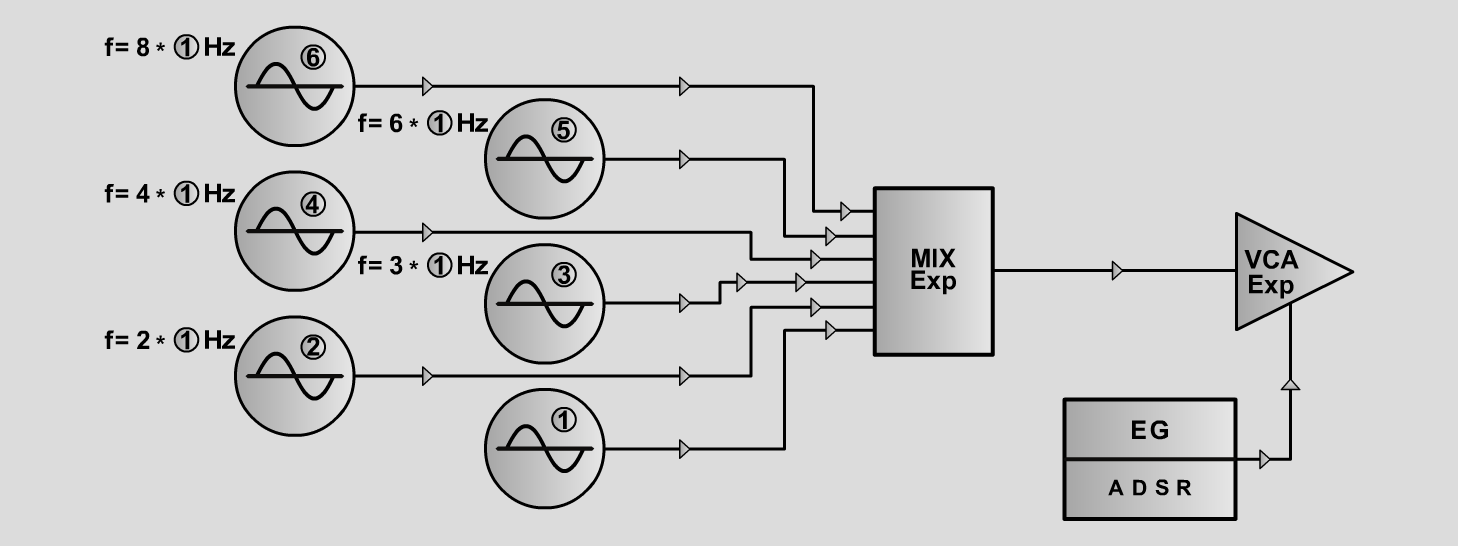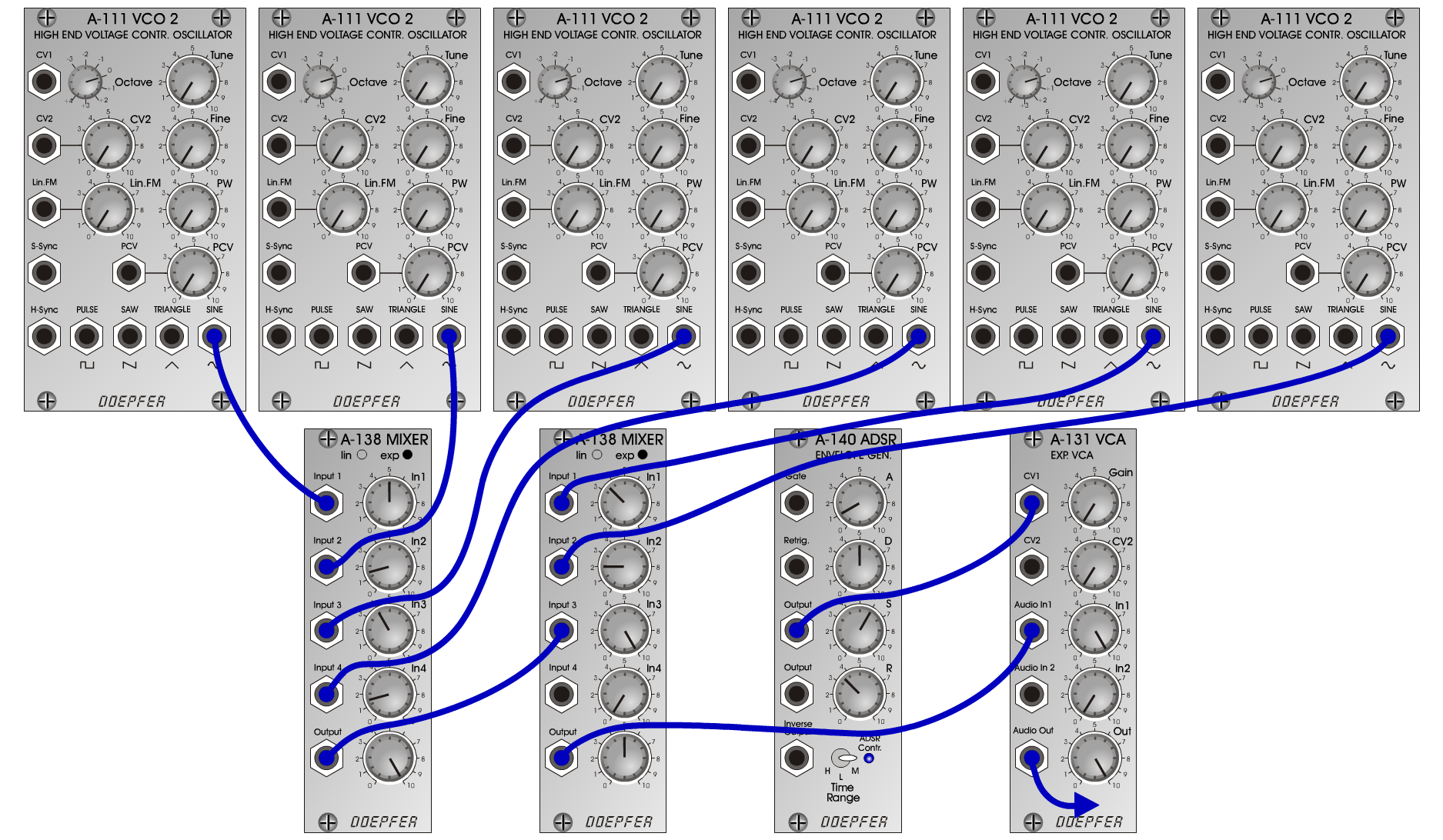Ganymede OrganKeyboardsD o e p f e r A - 100 Connections: Settings: Ganymede Organ Alpha A-111/1-4 (Sine) <=> A-138exp 1 (Input 1-4)  A-111/5-6 (Sine) <=> A-138exp 2 (Input 1-2)  A-138exp 1 (Output) <=> A-138exp 2 (Input 3)  A-138exp 2 (Output) <=> A-131 (Audio In)  A-140 (Output) <=> A-131 (CV 1) A-138exp 1 (In 1 = 5, In 2 = 1.5, In 3 = 4, In 4 = 1.5, Out = 10)  A-138exp 2 (In 1 = 3.5, In 2 = 2, In 3 = 10, Out = 5)  A-140 (A = 1, D = 5, S = 6, R = 3.5, Range = M)  A-131 (Gain = 0, Audio In 1 = 10, Audio Out = 10) Ganymede Organ Beta A-138exp 1 (In 1 = 5, In 2 = 3, In 3 = 2, In 4 = 3, Out = 10)  A-138exp 2 (In 1 = 1, In 2 = 0, In 3 = 10, Out = 5)  A-140 (A = 1, D = 5, S = 6, R = 3.5, Range = M)  A-131 (Gain = 0, Audio In 1 = 10, Audio Out = 10) Ganymede Organ Gamma A-138exp 1 (In 1 = 5, In 2 = 3.5, In 3 = 2.5, In 4 = 4.5, Out = 10)  A-138exp 2 (In 1 = 5, In 2 = 0, In 3 = 10, Out = 5)  A-140 (A = 2, D = 5, S = 6, R = 3.5, Range = M)  A-131 (Gain = 0, Audio In 1 = 10, Audio Out = 10) Ganymede Organ Delta A-138exp 1 (In 1 = 2, In 2 = 1.5, In 3 = 2.5, In 4 = 1.5, Out = 10)  A-138exp 2 (In 1 = 4.5, In 2 = 4.5, In 3 = 10, Out = 5)  A-140 (A = 3, D = 5, S = 6, R = 3.5, Range = M)  A-131 (Gain = 0, Audio In 1 = 10, Audio Out = 10) Ganymede Organ Epsilon A-138exp 1 (In 1 = 4.5, In 2 = 2, In 3 = 3, In 4 = 1, Out = 10)  A-138exp 2 (In 1 = 2, In 2 = 1, In 3 = 10, Out = 5)  A-140 (A = 7, D = 6, S = 6, R = 7, Range = M)  A-131 (Gain = 0, Audio In 1 = 10, Audio Out = 10) Ganymede Organ Zeta A-138exp 1 (In 1 = 5, In 2 = 2, In 3 = 2, In 4 = 0, Out = 10)  A-138exp 2 (In 1 = 1, In 2 = 1, In 3 = 10, Out = 5)  A-140 (A = 1, D = 5, S = 6, R = 3.5, Range = M)  A-131 (Gain = 0, Audio In 1 = 10, Audio Out = 10) Ganymede Organ Eta A-138exp 1 (In 1 = 1, In 2 = 4, In 3 = 5, In 4 = 4, Out = 10)  A-138exp 2 (In 1 = 2, In 2 = 1, In 3 = 10, Out = 5)  A-140 (A = 1, D = 5, S = 6, R = 3.5, Range = M)  A-131 (Gain = 0, Audio In 1 = 10, Audio Out = 10) Ganymede Organ Theta A-138exp 1 (In 1 = 5, In 2 = 1, In 3 = 2, In 4 = 1, Out = 10)  A-138exp 2 (In 1 = 4, In 2 = 5, In 3 = 10, Out = 5)  A-140 (A = 1, D = 5, S = 6, R = 3.5, Range = M)  A-131 (Gain = 0, Audio In 1 = 10, Audio Out = 10) Notes: Tune the VCOs for example: VCO 1= 261.63 Hz, VCO 2= 523.25 Hz, VCO 3= 784 Hz, VCO 4= 1046.50 Hz, VCO 5= 1568 Hz, VCO 6= 2093 Hz. Josef MuellerSound samples Pieces from Bach: Toccata & Fuge D minor (BWV 565) - with reverb Ganymede Organ Piece (all Organs) Alpha Piece Alpha (220 Hz) Alpha (440 Hz) Beta Piece Beta (220 Hz) Beta (440 Hz) Gamma Piece Gamma (220 Hz) Gamma (440 Hz) Delta Piece Delta (220 Hz) Delta (440 Hz) Epsilon Piece Epsilon (220 Hz) Epsilon (440 Hz) Zeta Piece Zeta (220 Hz) Zeta (440 Hz) Eta Piece Eta (220 Hz) Eta (440 Hz) Theta Piece Theta (220 Hz) Theta (440 Hz)